/

### Marginal Tail Value-at-Risk (Marginal TVaR) when underlying distribution is multivariate normal

Suppose we have a set ofrisk factors which we can characterise by an-dimensional vector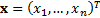. Suppose that the (active) exposures we have to these factors are characterised by another-dimensional vector,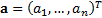. The aggregate exposure is then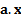.

The Value-at-Risk,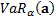, of the portfolio of exposures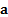at confidence level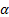, is defined as the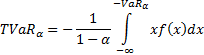The Marginal Tail Value-at-Risk,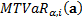, is the sensitivity of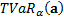to a small change in’th exposure. It is therefore: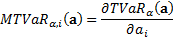In the case where the risk factors are multivariate normally distributed with mean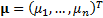and covariance matrix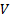whose elements are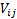we have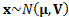and hence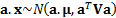. Hence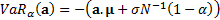.

Given the formula for the truncated first moments of a normal distribution we have: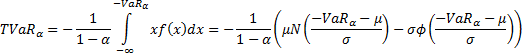where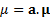,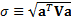,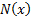is the (standard) normal cumulative distribution function and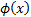is the (standard) normal probability density function.

Hence: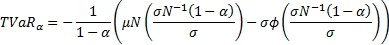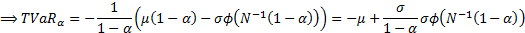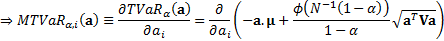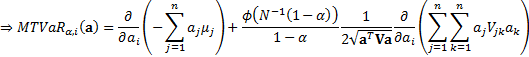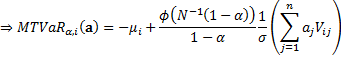The second of these terms can be expressed in terms of the correlation between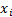andin a manner similar to Marginal VaR when underlying distribution is multivariate normal.

As risks arising from individual positions interact there is no universally agreed way of subdividing the overall risk into contributions from individual positions. However, a commonly used way is to define the Contribution to Tail Value-at-Risk,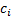, of the’th position,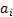to be as follows: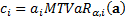Conveniently thethen sum to the overall VaR: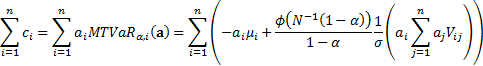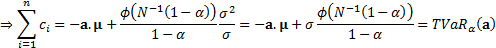The property that the contributions to risk add to the total risk is a generic feature of any risk measure that is (first-order) homogeneous, a property that Tail Value-at-Risk exhibits.